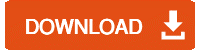# How to add Error Bars in Excel: Standard Deviation

How to add Error Bars in Excel: Standard Deviation? Imagine that if all data was perfect, absolute and complete. Although actually, it isn’t, Excel gives us some useful tools to convey margins of error and standard deviations.  If you work in a field that needs to reflect an accurate range of data error, you can add Error Bars to your charts and graphs.

## Let’s see how to add error bars in Excel?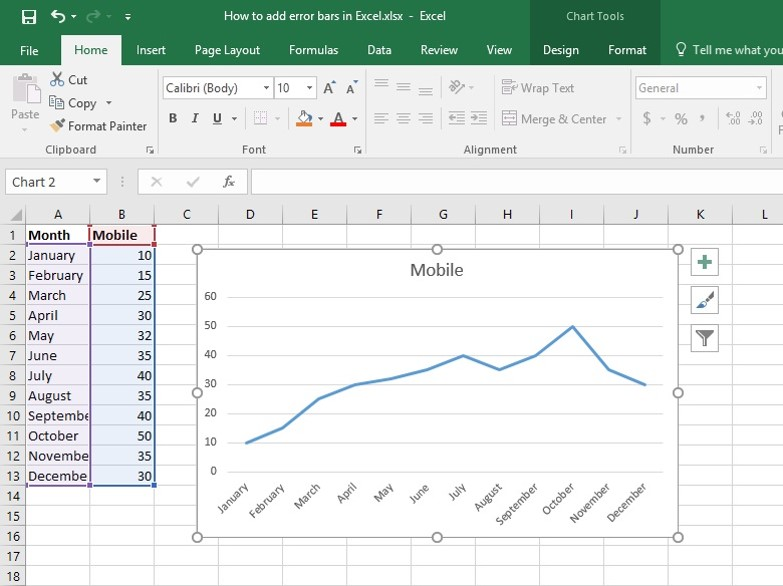Adding error bars in excel with this example, we have a linear graph which is showing the monthly sale of the mobile product of the shop.

Now we will add an Error Bar in this linear graph. You can learn this in our advanced excel course onlineExcel VBA Course (Beginner To Advanced)

If you want to be a master at Excel VBA Programming language for Excel 2007, then our Excel VBA macros tutorials will make it easier for you to access it in applications such as Microsoft Office. Come create your own Macros and rule in excel.

## How to add a standard bar in Excel or Standard Deviation?

Step 1: Click on the Chart.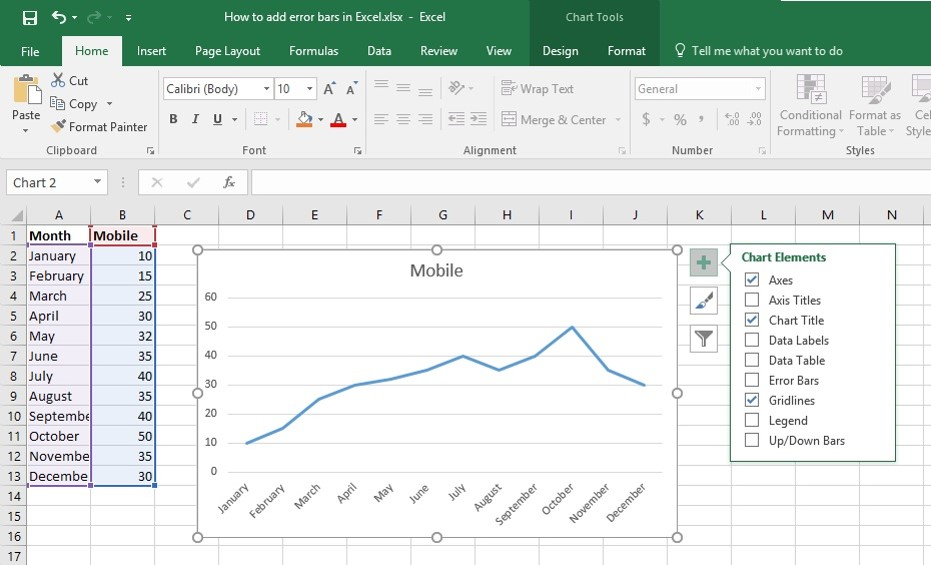Step 2: Click the Chart Elements Button to open the fly-out list of checkboxes.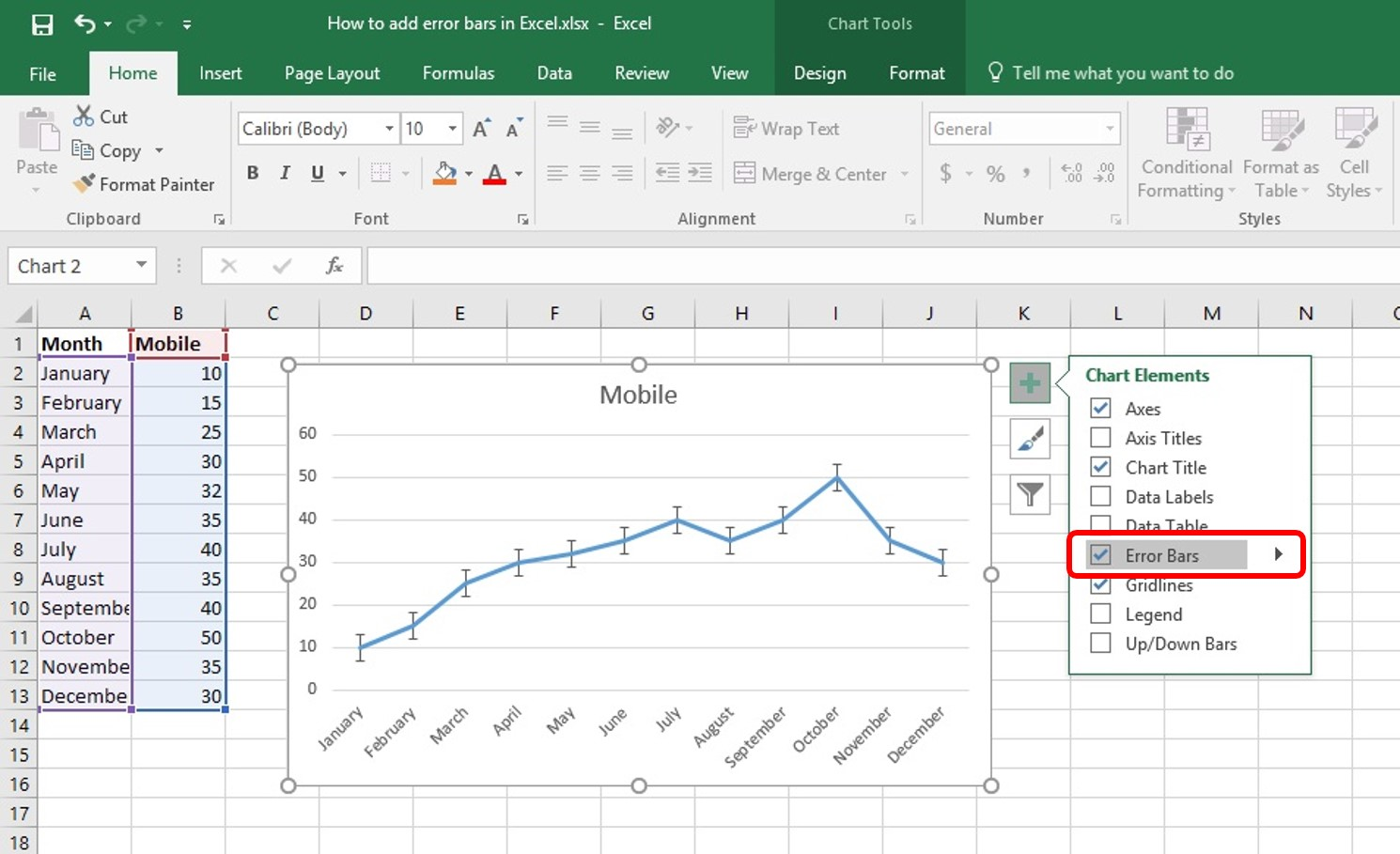Step 3: Put a check in the Error Bars checkbox. Then the graph will be looked like above picture.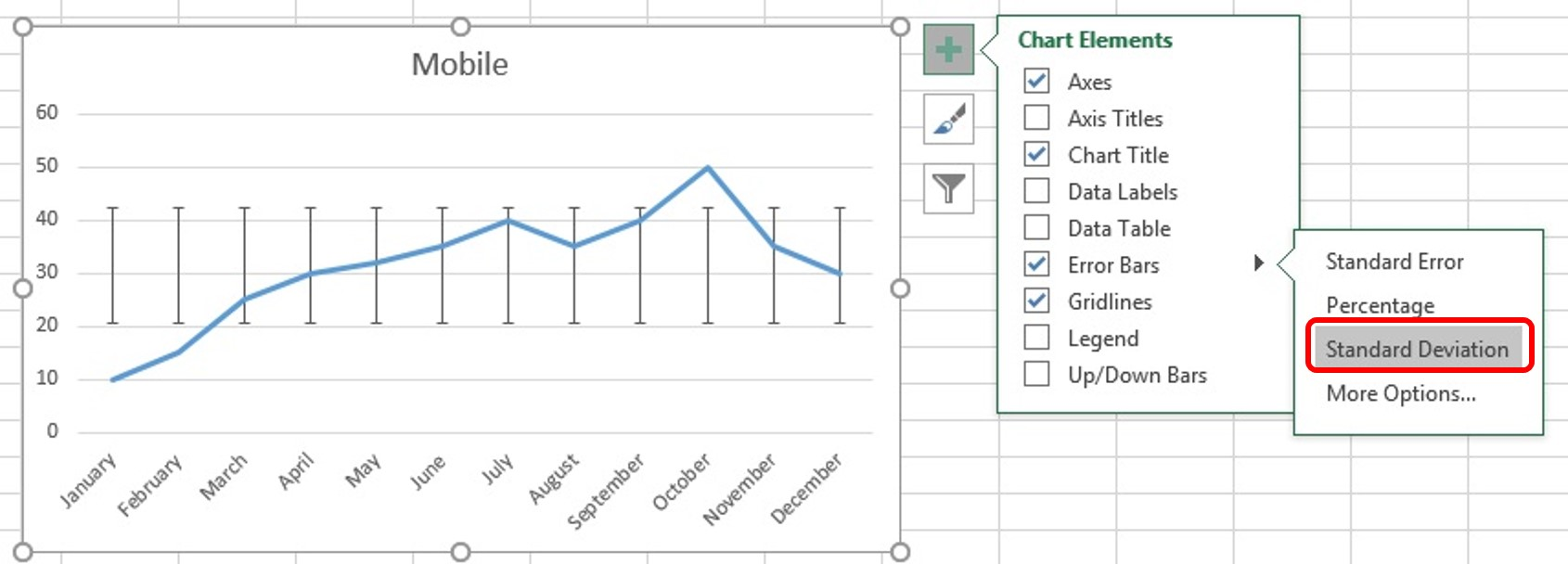Step 4: Click the arrow beside the Error Bars checkbox to choose from common error types. There are 3 types of Error:

1. Standard Error: To display standard error amount for all values.
2. Percentage: To specify a percentage error range and Excel will calculate the error amount for each value. Default percentage is 5%.
3. Standard Deviation: To display standard deviation error amount for all values. Resulting X &Y error bars will be the same size and won’t vary with each value.Recommended Courses

Learn how to insert an image into cells in Excel or you can learn such more tricks. Learn More

Create Dynamic Graphs & Excel Presentation. Also, Learn how to create Data Analytic Chart & Graph. Learn MoreExcel uses the above equation to calculate Standard Deviation Amount. Here, s = series number I = point number in series s m = number of series for point y in the chart n = number of points in each series y is = data value of series s and i the point n y = total number of data values in all series M = arithmetic mean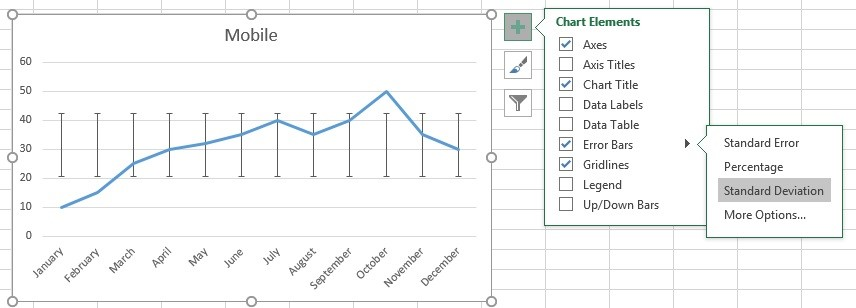Step 5: Click Standard Deviation Error from the Error list of Error bars. Now our graph will be looked like above picture.

So We have added error bar with Standard Deviation in our linear graph! Now let’s get some Tips!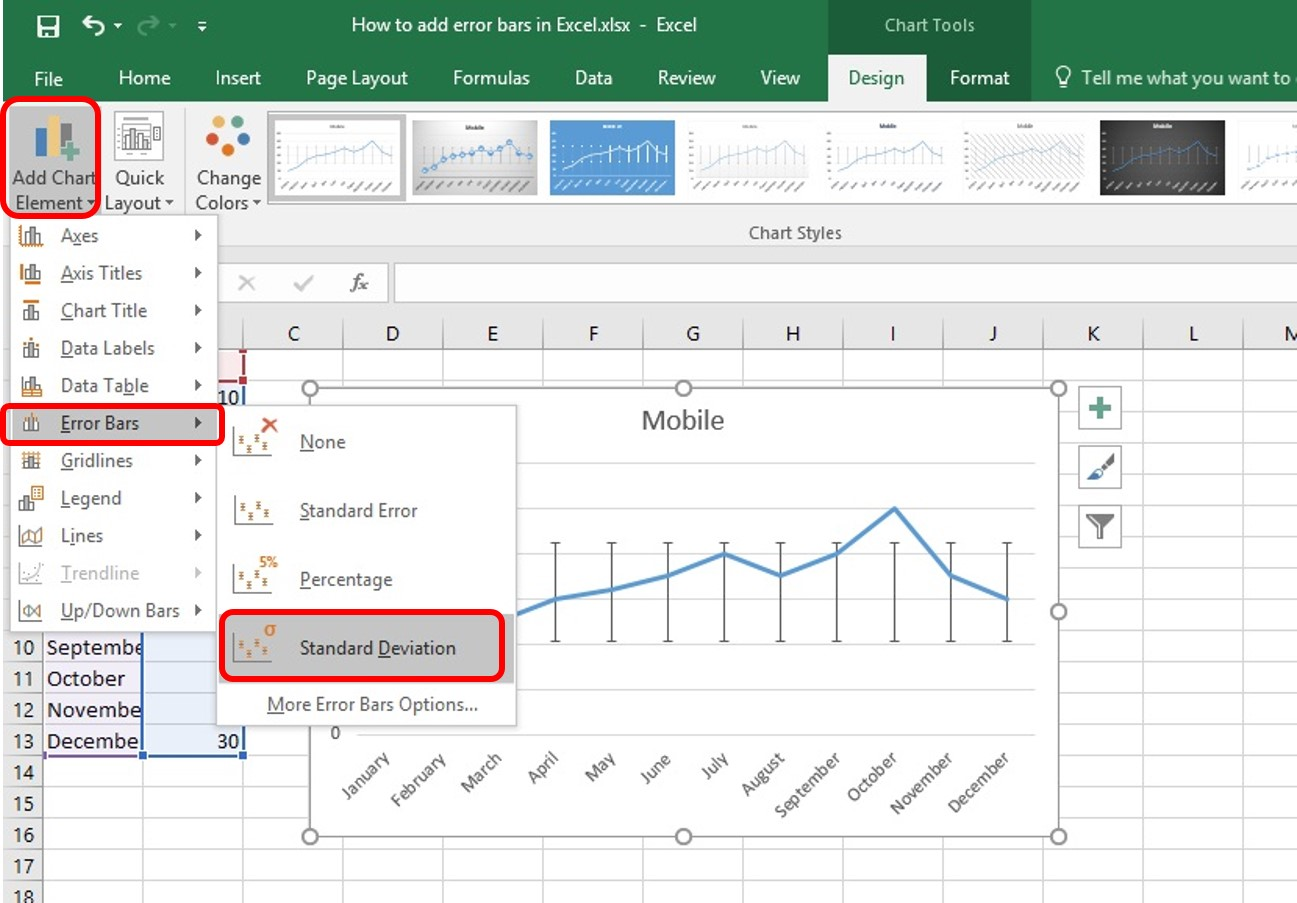Tips 1: We can also turn on Error bars from the Add Chart Element drop-down button on the Design tab under the Chart Tools contextual tab.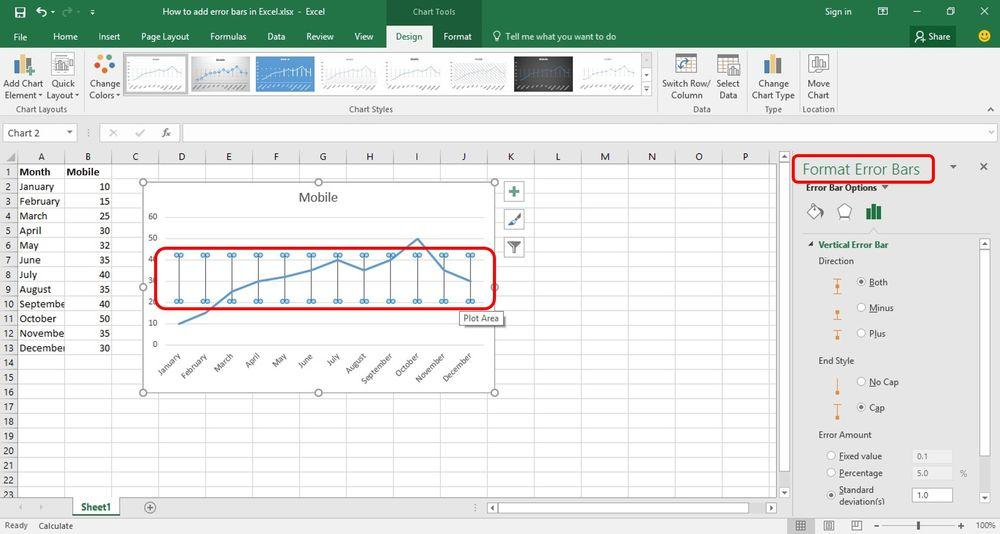Tips 2: Customize Error Bar Settings

Double click on any Error Bar inside the graph. It will open the Format Error Bars Task Pane with many options to customize Error Bar. You can Change the color, Direction, End Style and much more. So, Now we have learned about how to add Error Bars with Standard Deviation in Graph with some tips!

Learn how to insert image into cells in Excel or you can learn such more tricks. Learn More

Create Dynamic Graphs & Excel Presentation. Also, Learn how to create Data Analytic Chart & Graph. Learn More

The direction of the error bars depends on the type of chart you’re using. Scatter charts can show both horizontal and vertical error bars. You can remove either of these error bars by selecting them, and then be pressing Delete.

The ease with which our tutorials provide students with all the necessary information enables our students to efficiently and effectively get the most out of Excel, the world’s most popular software for any kind of business.

Download this blog as a pdf: